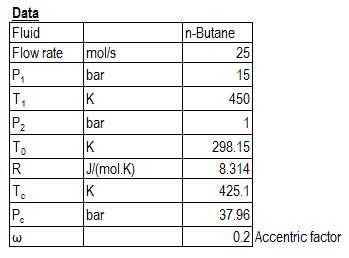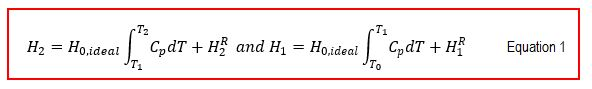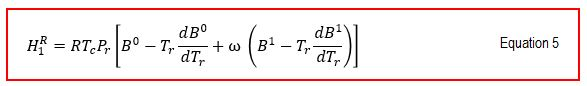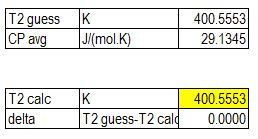# Estimating Exit Temperature in Throttling Process (Free Calculation Spreadsheet)

When fluid flow through a restriction, for example valve or orifice, pressure drop occurs adiabatically. If changes in kinetic or potential energy are negligible, which causes no change in enthalpy at the inlet and at the outlet, then we have ΔH = 0. Some properties of throttling process are expressed in figure below.

• In an ideal gas, enthalpy is a function of temperature only, and temperature remains constant
• At moderate temperature and pressure, throttling causes a drop in temperature for most real gases
• If wet steam is throttled to a considerably low pressure, the liquid evaporates and the steam becomes superheated
• The throttling of saturated liquid causes vaporization (or flashing) of some of the liquid, which produces saturated vapor and liquid

In this post, I want to share to you how to estimate exit temperature of gas after throttling and compare it with process simulation result.

This example is taken from Nonequibilibrium Thermodynamics: Transport and Rate Processes in Physical, Chemical, and Biological Systems.

Example:

n-Butane gas with a flow rate of 25 mol/s is throttled from 15 bar and 450 K to 1 bar in steady-state flow process. Determine the final temperature, assume that the surroundings are at 298.15 K.

Solution:

We assume that kinetic and potential energy are negligible.

We define the data as follow:Use Lee-Kesler generalized correlation for the reduced enthalpy estimations in a throttling process.

The reduced properties lead to enthalpies.where:By using the throttling property of ΔH = 0 and Equation 1, we getAt outlet condition, the n-butane gas is ideal, and Hence HR2 = 0, therefore Equation 2 becomesReduced properties and heat capacity:From Equation 4, we get:

Tr = 1.06

Pr = 0.40

Using the generalized equation, we havewhere

From Equation5,6 and Equation 7 we get:Since we need final temperature to estimate average heat capacity, we make an initial guess of final temperature and use iteration until guessed final temperature is the same as calculated final temperature.We get final temperature due to throttling process is 400 K.

If you want to learn how to estimate final temperature of throttling process, I share the spreadsheet of above calculation. I hope you find this post useful.

References:

Nonequilibrium Thermodynamics, 2nd Edition, Transport and Rate Processes in Physical, Chemical and Biological Systems

## 2 thoughts to “Estimating Exit Temperature in Throttling Process (Free Calculation Spreadsheet)”

1.Dede Arpendo says:

Sangatt menarik bu rifka, saya ingin bertanya kalo untuk fraksi naphta/kero apakah perhitungan tsb bisa diterapkan ?

1.Rifka Aisyah says:

Bisa De